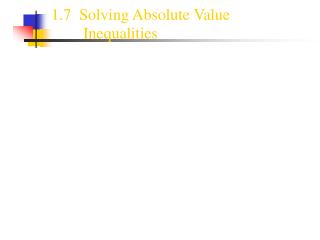DownloadDownload Presentation1.7 Solving Absolute Value Inequalities

# 1.7 Solving Absolute Value Inequalities

Télécharger la présentation## 1.7 Solving Absolute Value Inequalities

- - - - - - - - - - - - - - - - - - - - - - - - - - - E N D - - - - - - - - - - - - - - - - - - - - - - - - - - -
##### Presentation Transcript

1. 1.7 Solving Absolute Value Inequalities

2. Review of the Steps to Solve a Compound Inequality: • Example: • This is a conjunctionbecause the two inequality statements are joined by the word “and”. • You must solve each part of the inequality. • The graph of the solution of the conjunction is the intersection of the two inequalities. Both conditions of the inequalities must be met. • In other words, the solution is wherever the two inequalities overlap. • If the solution does not overlap, there is no solution.

3. Review of the Steps to Solve a Compound Inequality: • Example: • This is a disjunctionbecause the two inequality statements are joined by the word “or”. • You must solve each part of the inequality. • The graph of the solution of the disjunction is the union of the two inequalities. Only one condition of the inequality must be met. • In other words, the solution will include each of the graphed lines. The graphs can go in opposite directions or towards each other, thus overlapping. • If the inequalities do overlap, the solution is all reals.

4. “and’’ Statements can be Written in Two Different Ways • 1. 8 < m + 6 < 14 • 2. 8 < m+6 and m+6 < 14 These inequalities can be solved using two methods.

5. 2 8 Method One Example : 8 < m + 6 < 14 Rewrite the compound inequality using the word “and”, then solve each inequality. 8 < m + 6 and m + 6 < 14 2 < m m < 8 m >2 and m < 8 2 < m < 8 Graph the solution:

6. 2 8 Method Two Example: 8 < m + 6 < 14 To solve the inequality, isolate the variable by subtracting 6 from all 3 parts. 8 < m + 6 < 14 -6 -6 -6 2 < m < 8 Graph the solution.

7. -4 3 ‘or’ Statements Example: x - 1 > 2 or x + 3 < -1 x > 3 x < -4 x < -4 or x >3 Graph the solution.

8. Solving an Absolute Value Inequality • Step 1: Rewrite the inequality as a conjunction or a disjunction. • If you have a you are working with a conjunction or an ‘and’ statement. Remember: “Less thand” • If you have ayou are working with a disjunction oran ‘or’ statement. Remember: “Greator” • Step 2: In the second equation you must negate the right hand side and reversethe direction of the inequality sign. • Solve as a compound inequality.

9. -4 3 Example 1: This is an ‘or’ statement. (Greator). Rewrite. In the 2nd inequality, reverse the inequality sign and negate the right side value. Solve each inequality. Graph the solution. • |2x + 1| > 7 • 2x + 1 > 7 or 2x + 1 >7 • 2x + 1 >7 or 2x + 1 <-7 • x > 3 or x < -4

10. 2 8 Example 2: This is an ‘and’ statement. (Less thand). Rewrite. In the 2nd inequality, reverse the inequality sign and negate the right side value. Solve each inequality. Graph the solution. • |x -5|< 3 • x -5< 3 and x -5< 3 • x -5< 3 and x -5> -3 • x < 8 and x > 2 • 2 < x < 8

11. Solve and Graph • 1) 4m - 5 > 7 or 4m - 5 < - 9 • 2) 3 < x - 2 < 7 • 3) |y – 3| > 1 • 4) |p + 2| < 6